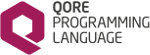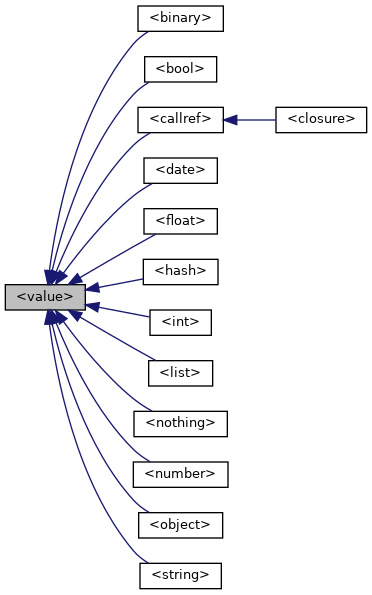Qore Programming Language Reference Manual  0.9.9
<value> Class Reference

Methods in this pseudo-class are available to be executed on any value type (even NOTHING); this is the root class for all pseudo-classes. More...

Inheritance diagram for Qore::<value>:[legend]

## Public Member Methods

bool callp ()
Returns False; this method is reimplemented in other types and will return True if the given expression is a callable value (ie closures or call references) More...

bool complexType ()
returns True if the value has a complex type, False if not More...

bool empty ()
Returns True; this method will be reimplemented in container types where it may return False. More...

string fullType ()
returns the full type name which differs from the simple type name in case of complex types and objects More...

bool intp ()
Returns False; this method is reimplemented in other types and will return True if the given expression can be converted to an integer. More...

AbstractIterator iterator ()
Returns an iterator object for the value; the default iterator object returned is SingleValueIterator. More...

int lsize ()
Returns 1; the return value of this method should give the list size of the value, which is normally 1 for non-lists (except for NOTHING where the size will be 0) and the number of the elements in the list for lists; this method will be reimplemented in other types where it may return other values. More...

int size ()
Returns zero; this method will be reimplemented in container types where it may return a non-zero value. More...

bool sizep ()
Returns True if the type can return a non-zero size (True for containers including binary objects and strings, False for everything else) More...

bool strp ()
Returns False; this method is reimplemented in other types and will return True if the given expression can be converted to a string. More...

bool toBool ()
Returns the boolean representation of the value; the default is False. More...

float toFloat ()
Returns the floating-point representation of the value; the default is 0.0. More...

int toInt ()
Returns the integer representation of the value; the default is 0. More...

number toNumber ()
Returns the arbitrary-precision numeric representation of the value; the default is 0. More...

string toString ()
Returns the string representation of the value; the default is an empty string. More...

string type ()
Returns the string type for the value. More...

int typeCode ()
Returns the type code for the value. More...

bool val ()
Returns False; this method is reimplemented in other types and will return True if the given expression has a non-empty value. More...

## Detailed Description

Methods in this pseudo-class are available to be executed on any value type (even NOTHING); this is the root class for all pseudo-classes.

## ◆ callp()

 bool ::callp ( )

Returns False; this method is reimplemented in other types and will return True if the given expression is a callable value (ie closures or call references)

Returns
False; this method is reimplemented in other types and will return True if the given expression is a callable value (ie closures or call references)
Code Flags:
CONSTANT
Example:
if (n.callp())
printf("the reuslt of calling the value: %y\n", n());

## ◆ complexType()

 bool ::complexType ( )

returns True if the value has a complex type, False if not

Code Flags:
CONSTANT
Example:
bool b = n.complexType();
Returns
True if the value has a complex type, False if not; if this pseudo-method returns True, then the return value of <value>::fullType() will differ from that of <value>::type()

Complex types are:

Since
Qore 0.8.13

## ◆ empty()

 bool ::empty ( )

Returns True; this method will be reimplemented in container types where it may return False.

This pseudo-method will return False in all non-container types; use <value>::val() to check if a generic expression that might not be a container type has a value instead.

Returns
True; this method will be reimplemented in container types where it may return False
Code Flags:
CONSTANT
Example:
int i = 100;
if (i.empty())
printf("%y: is empty (probably because it's a value of type %y)\n", i, i.type());
<value>::val()

## ◆ fullType()

 string ::fullType ( )

returns the full type name which differs from the simple type name in case of complex types and objects

Code Flags:
CONSTANT
Example:
hash <string, int> h();
# returns "hash<string, int>"
string str = n.fullType();
Mutex m();
# returns "object<Mutex>"
str = m.fullType();
Returns
the full type name which differs from the simple type name in case of complex types and objects; for simple types the return value is the same as for <value>::type()

Complex types are:

• hashdecl hashes: the complex type description is returned (ex: `"hash<MyHash>"`)
• complex hashes: the complex type description is returned (ex: `"hash<string, int>"`)
• complex lists: the complex type description is returned (ex: `"list<int>"`)
• objects: the complex type description is returned (ex: `"object<MyClass>"`; see Class-Specific Type Declaration)
Since
Qore 0.8.13

## ◆ intp()

 bool ::intp ( )

Returns False; this method is reimplemented in other types and will return True if the given expression can be converted to an integer.

Returns
False; this method is reimplemented in other types and will return True if the given expression can be converted to an integer
Code Flags:
CONSTANT
Example:
if (n.intp())
printf("%y: can be converted to an integer: %d\n", n, int(n));
<string>::intp() for an example of an implementation of this method where the result depends on the value and not the type

## ◆ iterator()

 AbstractIterator ::iterator ( )

Returns an iterator object for the value; the default iterator object returned is SingleValueIterator.

Returns
an iterator object for the value; the default iterator object returned is SingleValueIterator
Code Flags:
CONSTANT
Example:
map printf("+ %y\n", \$1), v.iterator();
Since
Qore 0.8.6

## ◆ lsize()

 int ::lsize ( )

Returns 1; the return value of this method should give the list size of the value, which is normally 1 for non-lists (except for NOTHING where the size will be 0) and the number of the elements in the list for lists; this method will be reimplemented in other types where it may return other values.

Returns
1; the return value of this method should give the list size of the value, which is normally 1 for non-lists (except for NOTHING where the size will be 0) and the number of the elements in the list for lists; this method will be reimplemented in other types where it may return other values
Code Flags:
CONSTANT
Example:
printf("iterating %d element%s\n", val.lsize(), val.lsize() == 1 ? "" : "s");
foreach any element in (val) {
do_something(element);
}

## ◆ size()

 int ::size ( )

Returns zero; this method will be reimplemented in container types where it may return a non-zero value.

This pseudo-method will return 0 in all non-container types; use <value>::val() to check if a generic expression that might not be a container type has a value instead.

Returns
zero; this method will be reimplemented in container types where it may return a non-zero value
Code Flags:
CONSTANT
Example:
int i = 100;
if (!i.size())
printf("%y: has size 0 (probably because it's a value of type %y)\n", i, i.type());

## ◆ sizep()

 bool ::sizep ( )

Returns True if the type can return a non-zero size (True for containers including binary objects and strings, False for everything else)

Code Flags:
CONSTANT
Returns
True if the type can return a non-zero size (True for containers including binary objects and strings, False for everything else)
<value>::size()
Since
Qore 0.8.9

## ◆ strp()

 bool ::strp ( )

Returns False; this method is reimplemented in other types and will return True if the given expression can be converted to a string.

Returns
False; this method is reimplemented in other types and will return True if the given expression can be converted to a string
Code Flags:
CONSTANT
Example:
if (n.strp())
printf("%y: can be converted to a string: '%s'\n", n, string(n));

## ◆ toBool()

 bool ::toBool ( )

Returns the boolean representation of the value; the default is False.

Code Flags:
CONSTANT
Returns
the boolean representation of the value; the default is False
Note
if <value>::intp() returns True, then the value can also be converted to a boolean value
Since
Qore 0.8.6

## ◆ toFloat()

 float ::toFloat ( )

Returns the floating-point representation of the value; the default is 0.0.

Code Flags:
CONSTANT
Returns
the floating-point representation of the value; the default is 0.0
Note
if <value>::intp() returns True, then the value can also be converted to a floating-point value
Since
Qore 0.8.6

## ◆ toInt()

 int ::toInt ( )

Returns the integer representation of the value; the default is 0.

Code Flags:
CONSTANT

Use <value>::intp() to determine if the current value can be converted to an integer

Returns
the integer representation of the value; the default is 0
Since
Qore 0.8.6

## ◆ toNumber()

 number ::toNumber ( )

Returns the arbitrary-precision numeric representation of the value; the default is 0.

Code Flags:
CONSTANT
Returns
the integer representation of the value; the default is 0
Note
if <value>::intp() returns True, then the value can also be converted to an arbitrary-precision numeric value
Since
Qore 0.8.8

## ◆ toString()

 string ::toString ( )

Returns the string representation of the value; the default is an empty string.

Code Flags:
CONSTANT

Use <value>::strp() to determine if the current value can be converted to a string

Returns
the string representation of the value; the default is an empty string
Note
check pseudo-classes for each type for other variants of this method; some may take arguments to affect the output format
Since
Qore 0.8.6

## ◆ type()

 string ::type ( )

Returns the string type for the value.

Code Flags:
CONSTANT
Example:
string t = n.type();
Returns
the string type for the value; see String Type Constants for possible return values for each type
Note
It is faster and more efficient to use <value>::typeCode() for comparing data types

## ◆ typeCode()

 int ::typeCode ( )

Returns the type code for the value.

Code Flags:
CONSTANT
Example:
switch (v.typeCode()) {
case NT_INT:
printf("%y: is an integer\n", v);
break;
case NT_DATE:
printf("%y: is a date\n", v);
break;
default:
printf("%y: is something else entirely\n", v);
break;
}

This method is recommended over <value>::type() or the type(any) or typename(any) functions for comparing data types as it is much faster and more efficient than the other alternatives (which work with string values instead of integer codes).

Returns
the type code for the value; see Type Code Constants for possible return values for each type

## ◆ val()

 bool ::val ( )

Returns False; this method is reimplemented in other types and will return True if the given expression has a non-empty value.

Returns
False; this method is reimplemented in other types and will return True if the given expression has a non-empty value
Code Flags:
CONSTANT
Example:
if (n.val())
printf("%y: has a non-empty value\n", n);
Rules:
 Type Rule int, float, number False if zero, True if non-zero string False if empty, True if not empty list False if empty, True if not empty hash False if empty, True if not empty object always True with a valid object code always True NOTHING always False NULL always False
Example Values:
 Example Result 0.val() False 1.val() True "".val() False (empty string) "0".val() True "string".val() True ().val() False (empty list) (0,).val() True {}.val() False (empty hash) ("a":0).val() True NOTHING.val() False NULL.val() False
Note
• Use Exists Operator (exists) to tell if an expression has a value or not
• This pseudo-method can be used to mimic perl's boolean evaluation when %strict-bool-eval is enabled; the return value of this method is the same as that of any value except `"0"` when evaluated in a boolean context when %perl-bool-eval is enabled (the default); note that `"0".val()` returns True while `boolean("0")` returns False in all cases.